NCERT Solutions for Class 8 Maths Chapter 9 Algebraic Expressions and Identities Ex 9.3 are part of NCERT Solutions for Class 8 Maths. Here we have given NCERT Solutions for Class 8 Maths Chapter 9 Algebraic Expressions and Identities Ex 9.3.

 Board CBSE Textbook NCERT Class Class 8 Subject Maths Chapter Chapter 9 Chapter Name Algebraic Expressions and Identities Exercise Ex 9.3 Number of Questions Solved 5 Category NCERT Solutions

## NCERT Solutions for Class 8 Maths Chapter 9 Algebraic Expressions and Identities Ex 9.3

Question 1.
Carry out the multiplication of the expressions in each of the following pairs:
(i) 4p, q + r
(ii) ab, a – b
(iii) $$a+b,\quad 7{ a }^{ 2 }{ b }^{ 2 }$$
(iv) $${ a }^{ 2 }-9,\quad 4a$$
(v) pq + qr + rp, 0
Solution.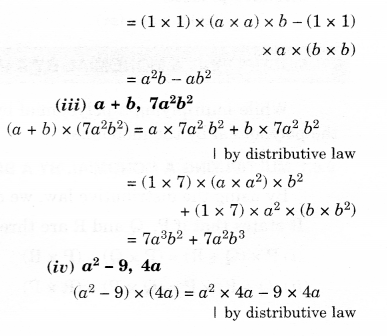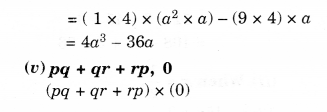Question 2.
Complete the tableSolution.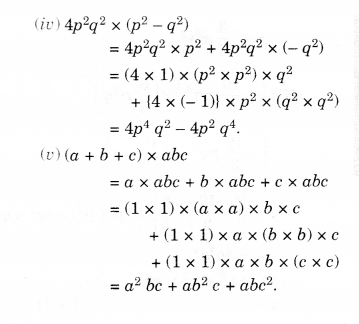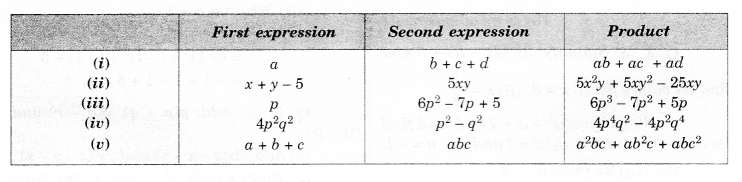Question 3.
Find the product:Solution.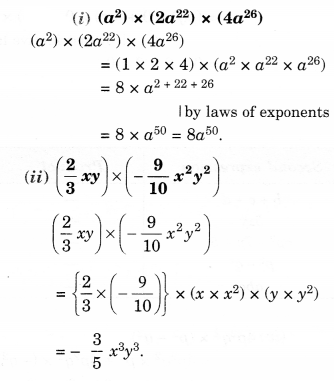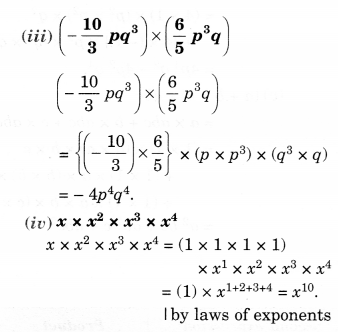Question 4.
(a) Simplify: 3x (4x – 5) + 3 and find its values for (i) x = 3, (ii) $$x=\frac { 1 }{ 2 }$$
(b) Simplify: $$a({ a }^{ 2 }+a+1)+5$$ and find its value for (i)a = 0, (ii)a = 1 and (iii) a = -1.
Solution.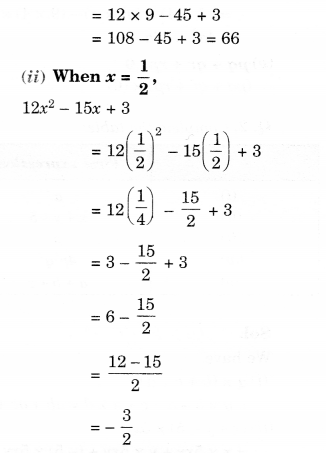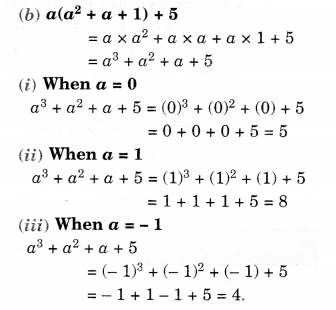Question 5.
(a) Add: p(p – q), q(q – r) and r(r -p)
(b) Add: 2x(z – x – y) and 2y (z – y – x)
(c) Subtract: 3l (l – 4m + 5n) from 4l (10n – 3m + 2l)
(d) Subtract: 3a(a + b + c) – 2b(a – b + c) from 4c(- a + b + c).
Solution.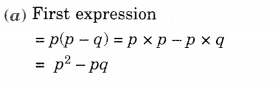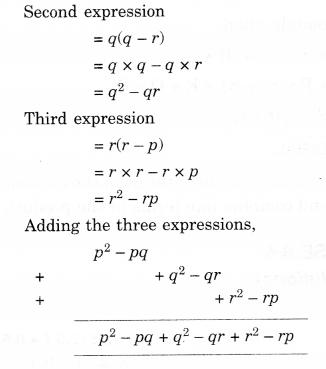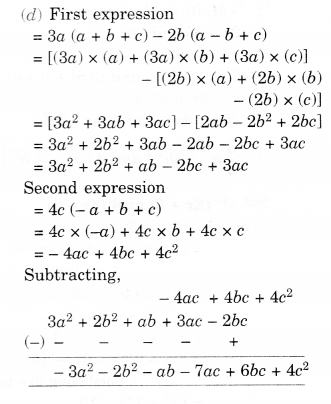We hope the NCERT Solutions for Class 8 Maths Chapter 9 Algebraic Expressions and Identities Ex 9.3 help you. If you have any query regarding NCERT Solutions for Class 8 Maths Chapter 9 Algebraic Expressions and Identities Ex 9.3, drop a comment below and we will get back to you at the earliest.